# 烧了微软10000张GPU，这伙人铁了心要砸大家的饭碗

2020年注定是不平凡的一年。除了新冠疫情之外，今年还可能是“天网元年”。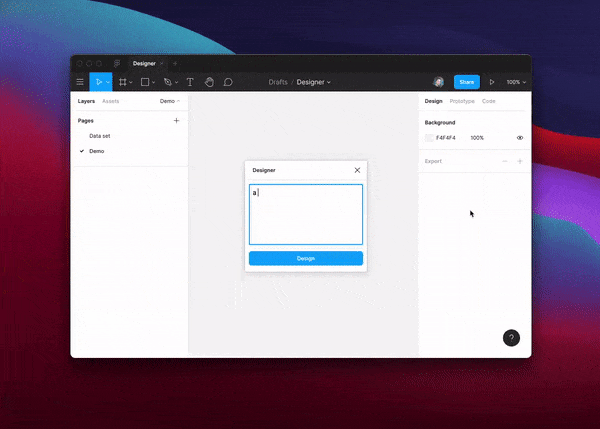# 全能选手GPT-3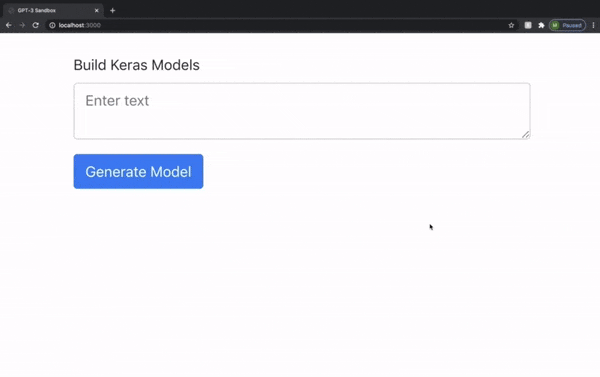GPT-3甚至连代码的注释都写好了，生怕你看不懂。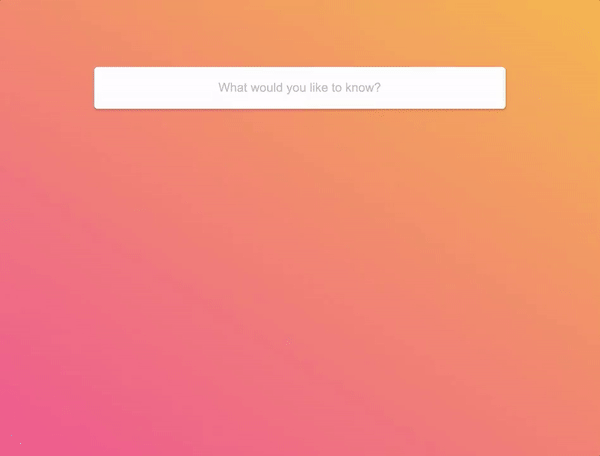GPT-3能根据描述生成网页CSS代码，稍加改动就能直接用在网页设计里。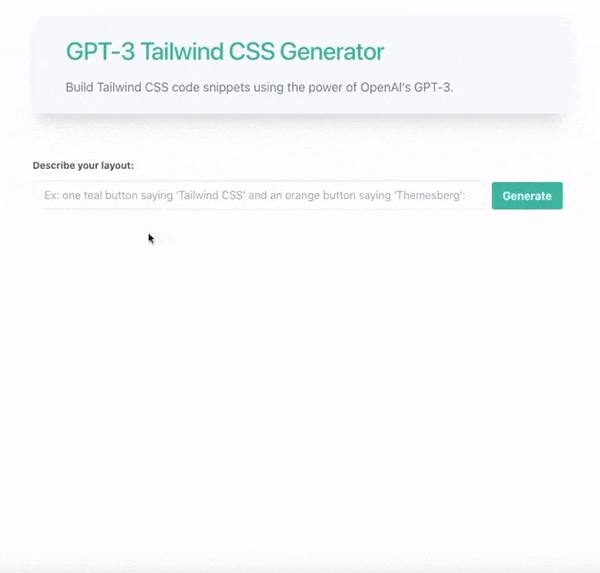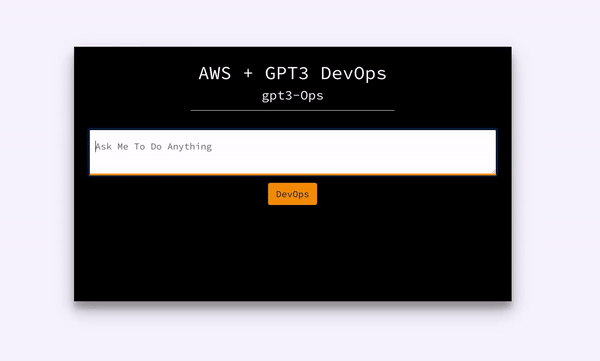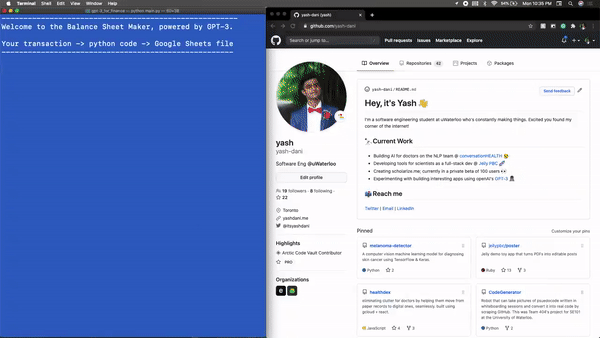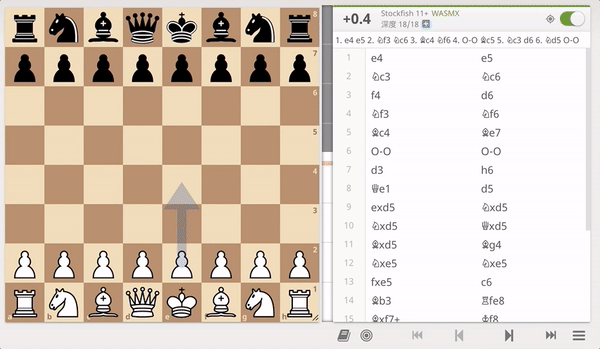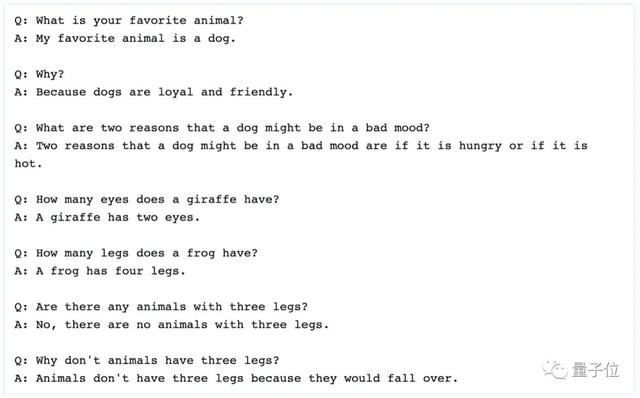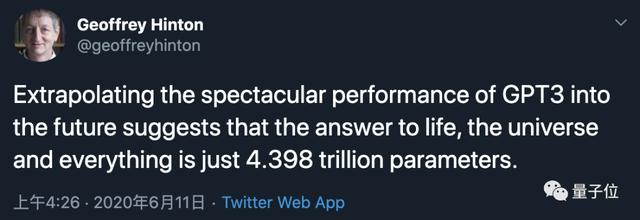# “钞能力”的GPT-3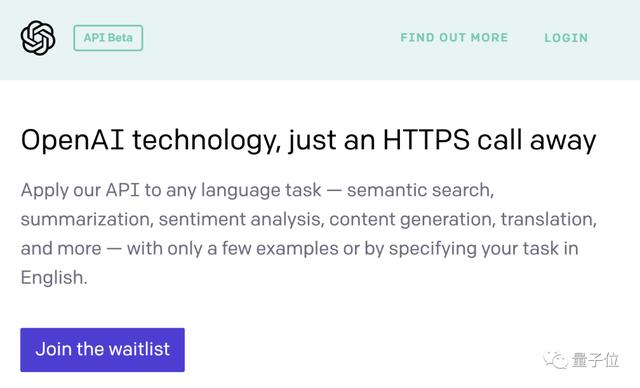GPT-3能力超强，一方面是因为在激烈竞争中各家NLP技术突飞猛进，更主要原因还是OpenAI够暴力、够多金。GPT-3采用了两阶段的思路。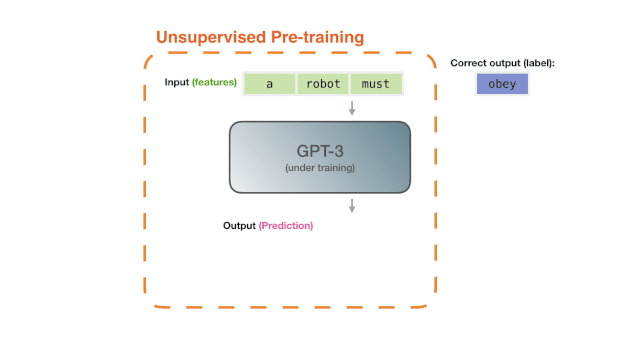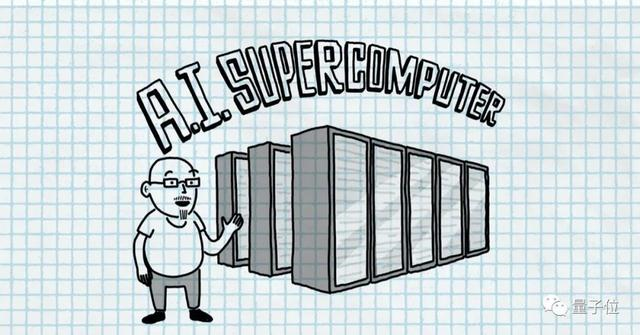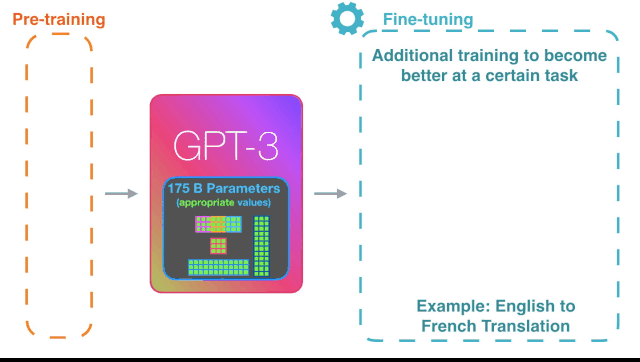# 颇具争议的GPT-3

GPT-3成为网红，当然也少不了网友的激烈讨论。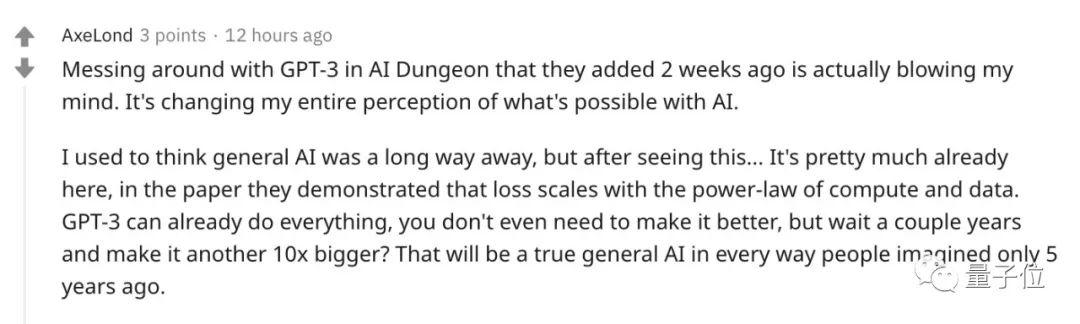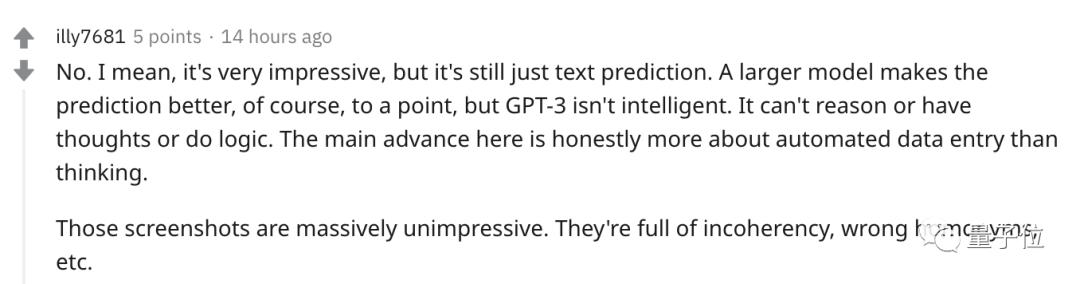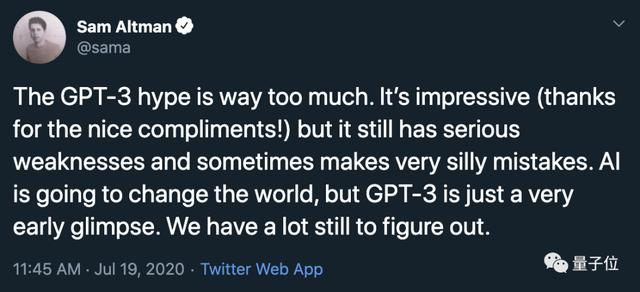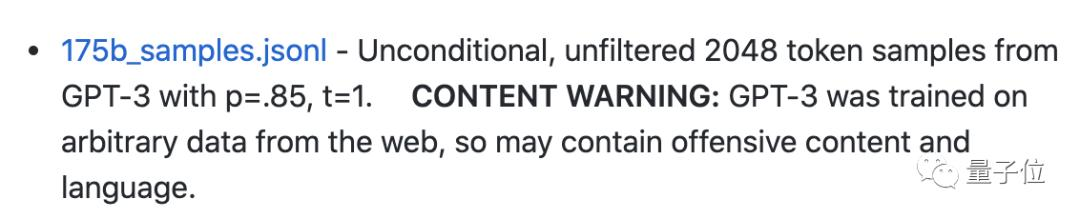△OpenAI官方提示，GPT-3接受网络内容训练，可能有令人反感的内容

# 如何申请试用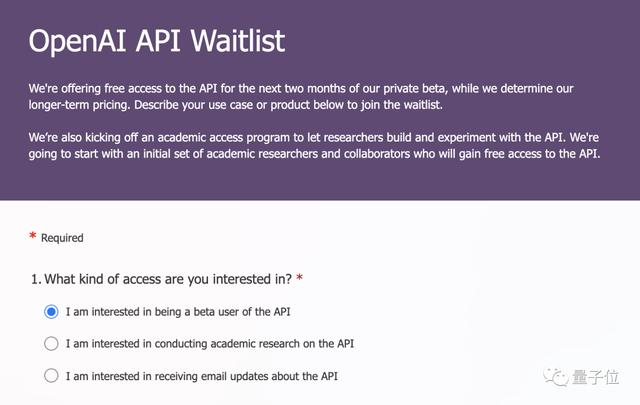# Construct GPT object and show some examples
gpt = GPT(engine="davinci",
temperature=0.5,
max_tokens=100)
gpt.add_example(Example('Two plus two equals four', '2 + 2 = 4'))
gpt.add_example(Example('The integral from zero to infinity', '\\int_0^{\\infty}'))
gpt.add_example(Example('The gradient of x squared plus two times x with respect to x', '\\nabla_x x^2 + 2x'))
gpt.add_example(Example('The log of two times x', '\\log{2x}'))
gpt.add_example(Example('x squared plus y squared plus equals z squared', 'x^2 + y^2 = z^2'))

# Define UI configuration
config = UIConfig(description="Text to equation",
button_text="Translate",
placeholder="x squared plus 2 times x")

demo_web_app(gpt, config)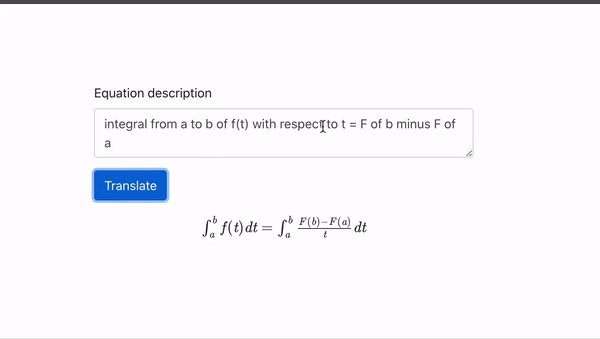https://blogs.microsoft.com/ai/openai-azure-supercomputer/
https://jalammar.github.io/how-gpt3-works-visualizations-animations/

OpenAI’s GPT-3 Language Generator Is Impressive, but Don’t Hold Your Breath for Skynet

GPT-3应用案例：
https://gpt3examples.com/

GPT-3沙箱：
https://github.com/shreyashankar/gpt3-sandbox

OpenAI API开发者工具包：
https://www.notion.so/API-Developer-Toolkit-49595ed6ffcd413e93ebff10d7e70fe7